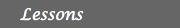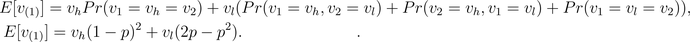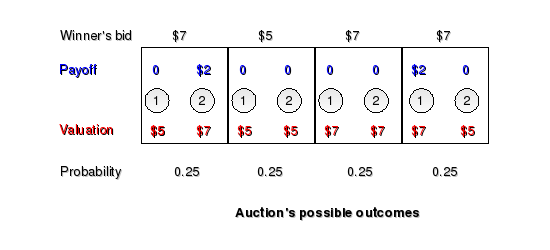Introduction1. Why study auctions? 2. The English and second-price sealed bid auctions 3. The Dutch and first-price  sealed bid auctions 4. The Revenue Equivalence  Theorem and further readingEnglish and second-price sealed-bid auctions

## English auction

The word "auction" is derived from the Latin augere, which means "to increase"
This is the most known kind of auction and is commonly used for selling goods, such as antiques and artwork. In this auction, the bidding price is raised until only one bidder remains. There are many ways this can be done, which essentially are equivalent. Either the auctioneer announces prices in an increasing manner, or bidders call their bids themselves, or bids are submitted online having the current highest bid posted at any time (see tangent). All of these methods have one common characteristic, at any point in time, all bidders know the current highest bid. We point out once more that we will assume in what follows hypotheses A1-A4 from the previous lesson.

## Example

Assume that an item is being offered in an English auction with only two risk-neutral bidders, bidders 1 and 2. The seller and bidder j (j=1,2) know that bidder i's valuation of the item is either vil with probability pi or vih with probability 1-pi (i=2,1). Suppose that 0< vil < vih for i=1,2. Assume further that the valuation of the item is independent among bidders (this corresponds to assumption A2, independent-private-values). Also, assume that v1l=v2l=:vl, v1h=v2h=:vh and p1=p2=:p (this corresponds to assumption A3, symmetric information). The payoff function for bidder i (=1,2), is either 0 if he never bids over bidder j (=2,1), or vl-(i's bid), or vh-(i's bid), depending on his/her valuation of the item, if he bids over bidder j (=2,1). Clearly the payoff function in this case is a function of the bids alone and assumption A4 holds (see figure). It seems to be clear that in this case bidders do not bid over their own valuation, and the winner of the auction is the bidder with the highest valuation who calls his/her bid first. The price he/she will pay for the item is equal to the valuation of the other bidder which is equal to the lowest valuation.Activity 1
Organize an English auction like the one explained above with two of your friends. You will act as the seller and they will act as two bidders. Explain the rules of the game to the bidders in a clear manner. What is the outcome of the auction? Does this outcome agree with the one explained before? How would the outcome have changed if some of the hypotheses A1-A4 are relaxed?

The outcome of the previous auction can be generalized to any English auction, assuming that A1-A4 hold. The highest valuation individual wins the bidding and pays a price equal to the valuation of his/her last remaining rival. However, the seller and the other bidders never know the true valuation of the winner of the auction. In what follows we will explain how to obtain, from the perspective of the seller, the average price paid by the winner of the auction. In order to do so we introduce the concept of order statistic.

## Order statistic

Suppose that X1, ..., XN are independent random quantities equally distributed, i.e for any value x the probability that Xi is less than or equal to x is the same for all i. We define the k-th order statistic, denoted by X(k), as the k-th smallest value among X1, ..., XN. For instance, in the example presented above if vi is the valuation of player i for i=1,2, and player 1 has a highest valuation than player 2, then v(1)=v2 and v(2)=v1.

## Expected price paid to the seller

Consider the example presented above. If vi is the valuation of player i for i=1,2, the price paid to the seller by the winner of the auction is equal to the lowest valuation, v(1). The expected value of this quantity is calculated below.Activity 2
1. Where have we used the independence of the valuations among bidders? Hint: The concept of independence is defined here.
2. If the seller considers that the valuation of each player can be determined by flipping a fair coin, with tails making the valuation equal to \$10 and heads equal to \$20. What is the expected price received by the seller in this case?

## Continuous distributions

In the example discussed above we have assumed that the distribution of the valuations is discrete, in the sense that the valuations are equal to specific values with positive probability. Nice results are achieved when one considers continuous distributions instead of discrete distributions. In this case the probability of having a valuation equal to x is equal to 0 for all x. What is possible to determine is the probability of having a valuation in a certain interval. The simplest of such distributions is the uniform distribution. We say that a random quantity X is uniformly distributed over the interval [a,b] if Pr(X≤ a)=0, Pr(X>b)=0 and for any x in [a,b], Pr(X≤x)=(x-a)/(b-a). For instance, it can be proved in this case that if the valuations of the bidders v1,v2 are independent and uniformly distributed on [0,1] then the expected amount received by the seller isA more general result is stated in lesson 4.

## The second-price sealed-bid auction

William Vickrey (1914-1996) was a Canadian professor of economics who was awarded with the Nobel Memorial Prize in Economics for his research on games with asymmetric information.
Under this auction, bidders submit sealed bids with the knowledge that the highest bidder wins the item but pays a price equal not to his/her own bid but the second highest bid (in case of a tie between m bidders, a random number between 1 and m is picked to decide the winner). This type of auction was created by William Vickrey, reason why is also known as the Vickrey's auction (see tangent). A modification of this type of auction is used by eBay's system and Google's and Yahoo!'s online advertisement programs.

## Example

Suppose that there are only two bidders in a second-price sealed-bid auction. Suppose that bidder 1's valuation of the item is \$5 and bidder 2's valuation is \$7. They both know their own valuation and also that the other bidder has a valuation of \$5 with probability 0.5 and \$7 with probability 0.5. If both bidders bid their own valuation, bidder 1 wins the item and has to pay \$5, a price equal to the lowest valuation (see figure). It turns out that bidding his/her own valuation is an equilibrium strategy for both bidders and therefore the amount of money received by the seller in this case is equal than the one obtained in an English auction.Activity 3
1. What is the expected price paid to seller in the auction described above?
2. Organize a second-price sealed bid-auction with two of your friends. Assume that their valuations satisfy the same properties as the ones listed in the example presented at the beginning of the lesson. You will act as the seller and they will act as two bidders. Explain the rules of the game to the bidders in a clear manner. What is the outcome of the auction? How would the outcome have changed if some of the hypotheses A1-A4 are relaxed?

## Incentive equilibrium

We claimed before that bidding their own valuation was an equilibrium strategy for every player in the second-price sealed-bid auction. The following reasoning shows why this holds. Suppose that bidder i submits a bid bi higher than its own valuation of the item, i.e bi>vi. Call M the maximum bid among the other bidders.
• If M < vi, then the bidder wins the item and his/her payoff would be equal to vi-M. The same payoff would be obtained by submitting his/her own valuation.
• If M > bi then the bidder does not win the auction and obtains a payoff of 0. The same payoff would be obtained by submitting his/her own valuation.
• Finally if, vi< M < bi, the bidder would win the auction but would have to pay a price higher than his/her own valuation. The payoff is less than 0, which is the one obtained by submitting his/her own valuation.
A similar argument shows that submitting the bidder's valuation is better than submitting bi< vi. This reasoning shows that revealing their own valuation is optimal for all bidders and the second-price sealed-bid auction is an incentive mechanism (see the revelation principle). This auction is different than the English auction in that the valuation of the winner is known to the seller. However, in both auctions the price paid for the item is the same and equal to the second highest valuation among bidders.

Activity 4
Explain why, in a second-price sealed-bid auction, it is not optimal to submit a bid less than the valuation of the item.